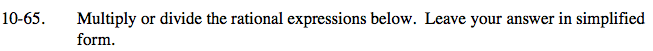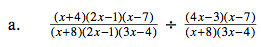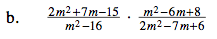### Home > CAAC > Chapter 10 > Lesson 10.2.2 > Problem10-65

10-65.See problem 10-30 for help multiplying and dividing fractional expressions.Remember that fractional division can also be expressed by multiplying by the reciprocal of a fraction.

$\frac{(x+4)(2x-1)(x-7)}{(x+8)(2x-1)(3x-4)}\cdot \frac{(x+8)(3x-4)}{(4x-3)(x-7)}$

Multiply the fractions and look for factors that simplify to one.

$\frac{(x+4)}{(4x-3)}$$\frac{(m+5)}{(m+4)}$